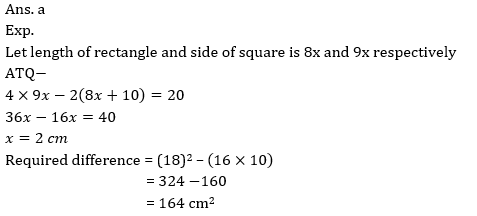# SBI PO Pre Quantitative Aptitude Quiz- 7

## SBI PO Pre Quantitative Aptitude Quiz 2022

Quantitative Aptitude is the most significant part of almost all competitive exams. Many candidates find difficulty in solving Quantitative Aptitude Questions. The only way to ace this section is to practice as many questions as possible. And, to help you practice more and more questions, we have come up with the SBI PO Pre Quantitative Aptitude Quiz. This SBI PO Pre Quantitative Aptitude Quiz is completely Free. This SBI PO Pre Quantitative Aptitude Quiz includes a variety of questions that are usually asked in preliminary and main exams of various banking exams. Candidates will be provided with a detailed solution for each question in this SBI PO Pre Quantitative Aptitude Quiz. This SBI PO Pre Quantitative Aptitude Quiz will assist candidates in better preparing for upcoming exams.

Directions (1-5): What should come in the place of question mark in the following number series?
1. 620, 632 , 608 , 644 , 596 , ?
(a) 536
(b) 556
(c) 656
(d) 646
(e) None of these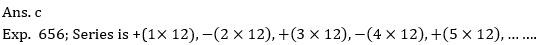2. 15 , 25 , 40 , 65 , ? , 195
(a) 115
(b) 90
(c) 105
(d) 120
(e) None of these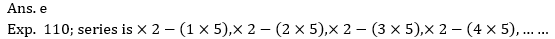3. 120 , 320 , ? , 2070 , 5195 , 13007.5
(a) 800
(b) 920
(c) 850
(d) 900
(e) 8204. 7 23 55 109 191 ?
(a) 307
(b) 317
(c) 333
(d) 343
(e) None of these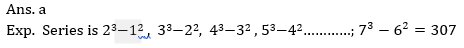5. 18 17 24 46 ?
(a) 85
(b) 118
(c) 112.5
(d) 92.5
(e) None of these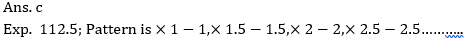6. If an article is sold at 25% discount at mark-price then loss percent is 10%. If the article is sold at marked price then what will be profit or loss percent?
(a) 50%
(b) 40%
(c) 30%
(d) 20%
(e) 25%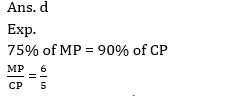7. Ramesh and Suresh can do a work in 18 days and 12 days respectively. Both are working together for x days and the Suresh left the work and Ramesh can do rest of the work in 8 days. Find the value of x is ?
(a) 6 days
(b) 4 days
(c) 8 days
(d) 12 days
(e) 10 days8. The ratio of numerical value of rate of interest and time period at a sum invested is 5 : 2 and men get 22.5% of invested sum as interest. Find at the same rate how much interest man will get on the sum of Rs. 1600 for two year ?
(a) 240 Rs.
(b) 180 Rs.
(c) 160 Rs.
(d) 120 Rs.
(e) 224 Rs.9. A and B invested Rs. 12600 together in a business. A invested for 8 months and B invested for 15 months, if A and B get profit in the ratio of 75 : 128, then find the amount invested by B ?
(a) 2400 Rs.
(b) 3000 Rs.
(c) 3600 Rs.
(d) 4800 Rs.
(e) 6400 Rs.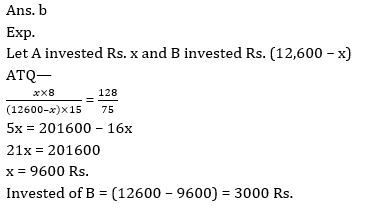10. The ratio between length of a rectangle and side of a square is 8 : 9. If breadth of rectangle is 10 cm and perimeter of square is 20 cm more than that of perimeter of rectangle, then find difference between area of square & that of rectangle?
(a) 164 cm2
(b) 172 cm2
(c) 174 cm2
(d) 156 cm2
(e) 144 cm2